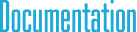#Quatf Class Reference

## Detailed Description

Quaternion class with float elements.Inheritance diagram for Quatf:

## Public Member Functions

Binary operators
Quat operator* (const Quat &q) const

Quat operator* (float f) const

Quat operator- (const Quat &q) const

Quat operator+ (const Quat &q) const

Set & Get value functions
void Zero ()

void Reset ()

void Set (float _s, const Point3< float > &_v)

void Set (float _s, float _x, float _y, float _z)

void Set (float *array)

void SetRotation (float angle, const Point3< float > &axis)

void SetRotation (float angle, float axisX, float axisY, float axisZ)

void GetValue (float *array)

float GetRotationAngle ()

Point3< float > GetRotationAxis ()

Length and Normalize functions
void Normalize ()

Quat GetNormalized () const

float LengthSquared () const

float Length () const

Matrix conversion functions
void ToMatrix3 (Matrix3< float > &m) const

Matrix3< float > ToMatrix3 () const

void ToMatrix4 (Matrix4< float > &m) const

Matrix4< float > ToMatrix4 () const

Unary operators
Quat operator- () const

Assignment operators
Quatoperator*= (const Quat &q)

Quatoperator*= (float f)

Quatoperator+= (const Quat &q)

Quatoperator-= (const Quat &q)

Test operators
int operator== (const Quat &q) const

int operator!= (const Quat &q) const

Vector rotations

Rotates the given vector using the quaternion. Note that the quaternion must be a unit quaternion.

Point3< float > GetRotatedVector (const Point3< float > &p)

void RotateVector (Point3< float > &p)

## Public Attributes

float s

Point3< float > v

## § Zero()

 void Zero ( )
inherited

Sets the scalar part to 1 and vector part to zero vector.

## § Reset()

 void Reset ( )
inherited

Sets the scalar part to 1 and vector part to zero vector.

## § Set() [1/3]

 void Set ( float _s, const Point3< float > & _v )
inherited

Sets the scalar part to 1 and vector part to zero vector.

## § Set() [2/3]

 void Set ( float _s, float _x, float _y, float _z )
inherited

Sets the scalar part to 1 and vector part to zero vector.

## § Set() [3/3]

 void Set ( float * array )
inherited

Sets the scalar part to 1 and vector part to zero vector.

## § SetRotation() [1/2]

 void SetRotation ( float angle, const Point3< float > & axis )
inherited

Sets the scalar part to 1 and vector part to zero vector.

## § SetRotation() [2/2]

 void SetRotation ( float angle, float axisX, float axisY, float axisZ )
inherited

Sets the scalar part to 1 and vector part to zero vector.

## § GetValue()

 void GetValue ( float * array )
inherited

Sets the scalar part to 1 and vector part to zero vector.

## § GetRotationAngle()

 float GetRotationAngle ( )
inherited

Returns rotation angle in radiants.

## § GetRotationAxis()

 Point3 GetRotationAxis ( )
inherited

Sets the scalar part to 1 and vector part to zero vector.

## § s

 float s
inherited

scaler part

## § v

 Point3 v
inherited

vector part